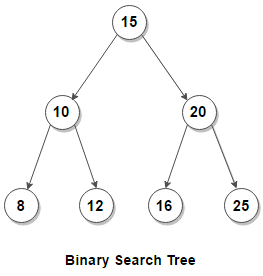# Insertion in BST | Recursive & Iterative Solution

A Binary Search Tree (BST) is a rooted binary tree, whose nodes each store a key (and optionally, an associated value) and each have two distinguished sub-trees, commonly denoted left and right. The tree should satisfy the BST property, which states that the key in each node must be greater than all keys stored in the left sub-tree, and not greater than all keys in the right sub-tree. Ideally, only unique values should be present in the tree.Binary search trees are a fundamental data structure used to construct more abstract data structures such as sets, multisets, and associative arrays (maps, multimaps, etc).

##### Insertion:

When looking for a place to insert a new key, we traverse the tree from root to leaf, making comparisons to keys stored in the nodes of the tree and deciding, based on the comparison, to continue searching in the left or right subtrees. In other words, we examine the root and recursively insert the new node to the left subtree if its key is less than that of the root, or the right subtree if its key is greater than or equal to the root.## C++

We can modify the above solution to return the root node from the function instead of passing it by reference.

## Java

##### Iterative Version –

Another way to explain the insertion is that in order to insert a new node in the tree, its key is first compared with that of the root. If its key is less than the root’s, it is then compared with the key of the root’s left child. If its key is greater, it is compared with the root’s right child. This process continues, until the new node is compared with a leaf node, and then it is added as this node’s right or left child, depending on its key: if the key is less than the leaf’s key, then it is inserted as the leaf’s left child, otherwise as the leaf’s right child.

## Java

The time complexity of above solution is O(h) where h is the height of the BST. The height of the BST in worst case is as much as number of keys in BST. The worst case happens when given keys are sorted in ascending or descending order and we get a skewed tree (all the nodes except leaf have one and only one child).

For a height balanced BSTs, with each comparison we skip about half of the tree, so that each insertion operation takes time proportional to the logarithm of the number of items n stored in the tree i.e. log2n. This is much better than the linear time required to find items by key in an (unsorted) array, but slower than the corresponding operations on hash tables.

Deletion from BST

Exercise: Modify the solution to construct a height balanced BST.(1 votes, average: 5.00 out of 5)Loading...